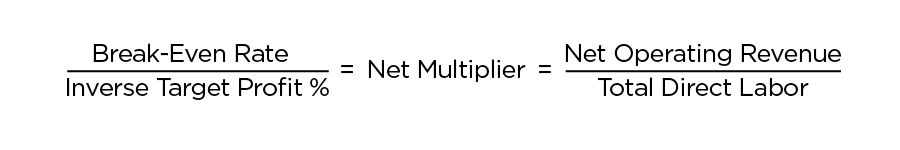•Hi Valerie,

Thank you for your response. I did see this post before. But when I try to derive the formula myself, it still did not make sense as to these three questions. Could you elaborate on this formula? Thank you.

•Yuan, multiplying by the target profit constitutes a mark-up. Mark-up and profit are not the same thing. Here is a discussion where I explain the difference in more detail.

And here is a more cleanly formatted 'master formula' that you need to know heading into the exam. It's important that you understand the relationships, not just memorize the formula.•Hi Matthew,

Thank you for the explanation. I think I got this now. There are 2 issues here. First, "inverse" in this case is "1-target profit rate". So if the target profit is 20%, the inverse of it will be 80%, not 1/0.2

Second, the break-even + the target profit should be 100%, not 120%